Synchronization and Arbitration

# 10. Synchronization and Arbitration

The engineering discipline introduced in Chapter 8 allows us to design sequential circuits that are provably reliable so long as setup and hold time constraints -- our dynamic discipline -- is adhered to in the timing of input changes. This powerful engineering tool allows us to build arbitrarily large clocked digital systems from interconnected clocked components, but requires that the clocks of the component systems be synchronized for the components to communicate reliably. Where we can do so conveniently, we can satisfy the dynamic discipline by using a single clock throughout the system.

However, it is not always convenient -- or even possible -- to guarantee that inputs to a system are well behaved with respect to the system's clock; Section 9.2.6 discusses one such common application. These cases motivate us to explore the costs of violating the dynamic discipline, costs which are deceptively easy to underestimate and have left several decades of scars on the history of digital systems engineering.

One lesson from this chapter is the parallel roles of the static and dynamic disciplines in our digital abstraction.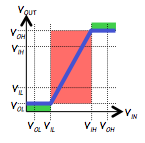The former enables us to map continuous voltages into the discrete values of our logic abstraction; its key feature is the exclusion of a range of voltages from the mapping, to avoid requiring logic to make difficult distinctions between voltages representing $0$ and $1$.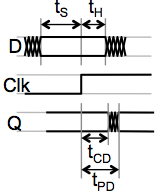The dynamic discipline enables a similar continuous-to-discrete mapping, but in the time domain. It allows us to partition continuous time into discrete clock cycles, reticulated by active clock edges. Like the static discipline, it avoids difficult mapping decisions by simply excluding a region of continuous time -- the setup and hold time window surrounding each active clock edge -- from the mapping. Thus these two cornerstone disciplines of our digital abstraction move us into a world of discrete values as well as discrete time.
An Input Synchronizer?

## 10.1. An Input Synchronizer?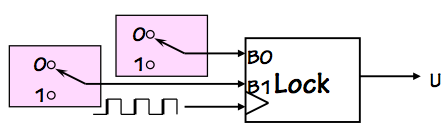Section 9.2.6 sketches a common motivation for dealing with asynchronous inputs, namely switch closures from buttons pressed by a human user as shown to the right. As the buttons may be pressed at arbitrary times, there is no guarantee that their changes will avoid the stated setup and hold time window specified by the clocked device. Typically in such situations we can assume that the clock frequency is fast with respect to button presses, and the timing of each actual switch closure comes at a random point during the clock cycle. If for example the clock period is $100 ns$ and the total $t_s+t_h$ specification for the clocked device is $10 ns$, we can expect that roughly $10$% of the button presses will not obey the dynamic discipline and thus potentially cause our clocked device to misbehave.Synchronizer
It is tempting to simply invent a fix in the form of a synchronizer component, to be used wherever it is necessary to deal with an asynchronous input. The synchronizer takes a local clock signal as well as an input $U(t)$ whose transitions are infrequent and asynchronous with respect to the clock. It produces, as output, a syncronous version $S(t)$ version of the $U(t)$ input that is well-behaved with respect to the clock: that is, $S(t)$ differs from $U(t)$ in that each transition has been delayed to avoid a specified setup and hold time window surrounding each active clock edge.

A workable synchronizer need only postpone troublesome transitions in its input until they are safely distant from active edges of the local clock. We can allow it to be conservative about choosing to delay transitions, allowing it to delay a few transitions that are just outside of the setup/hold time window. We can also be tolerant about the details of exactly when the postponed transitions appear in the $U(t)$ output, so long as they are synchronized with our clock; if our button press is neglected for an extra cycle of our gigahertz clock, the user is unlikely to notice. We might only require, for example, that each output transition occur within $N$ clock cycles of the corresponding input transition for some small constant $N$.

Given our tolerance for considerable sloppiness of this sort in the specifications of a synchronizer, it seems entirely plausible that such devices could be made to work reliably. Indeed, there was a time when major companies included synchronizers in their product lines. The remarkable and painful lesson learned during recent decades, however, is that synchronization is a much harder problem than it seems. It is, in fact, impossible to build a perfectly reliable synchronizer even given perfectly reliable components of the sort that have been developed here.

The difficulty is that, given a transition $T_B$ in the synchronizer input that is close to an active transition $T_C$ in the local clock, our synchronizer must decide whether $T_B$ comes before $T_C$. While our specifications allow the synchronizer's decisions to be imperfect, we require them to be made in a bounded amount of time -- a constraint that is problematic for fundamental reasons.

The Asynchronous Arbiter

## 10.2. The Asynchronous Arbiter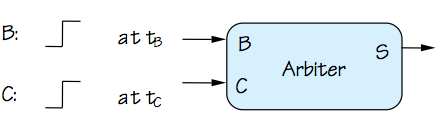Asynchronous Arbiter
The difficulty of knife-edge decision making in bounded time is modeled by the specifications of a hypothetical device called an asynchronous arbiter, shown to the right. The device takes two digital inputs $B$ and $C$, each of which makes a single $0 \rightarrow 1$ transition at times $T_B$ and $T_C$ respectively. The specifications for the arbiter involve a finite decision time $T_D$ and a finite allowable error $T_E$, and specify that the value of the output $S$ at time $T_C + T_D$ must be stable and valid no later than $T_D$ following the $T_C$ transition, and its value after time $T_C + T_D$ must be \begin{equation} S = \begin{cases} \mbox{valid 1}, & \mbox{if } t_B \lt t_C - t_E \\ \mbox{valid 0}, & \mbox{if } t_B \gt t_C + t_E \\ \mbox{valid 0 or 1}, & \mbox{otherwise} \end{cases} \end{equation} Many varients of this specification capture the same basic idea: the arbiter observes two asynchronous events, coded as positive transitions on input signals, and -- after a finite propagation delay $T_D$, reports which of the events came first. Again, our specifications seem entirely reasonable from an engineering standpoint: for close calls (events separated by less than $t_E$ seconds) we'll accept either decision as to the winner; we do, however, demand an unequivocal decision, in the form of a valid $0$ or $1$.

However reasonable our specification, it unfortunately cannot escape the surprising truth:

For no finite values of $T_E$ and $T_D$ is it possible to construct a perfectly reliable arbiter from reliable components that obey the laws of classical physics.
It took the digital community decades to accept this remarkable fact, seduced as we were by the digital abstraction. The difficulty, as we shall see, is the step of mapping a continuous variable (like voltage or time) to a discrete one in bounded time, the fundamental problem that motivates both our static and dynamic disciplines. Note that this problem arises from the continuous variables used in classical physics for underlying physical parameters; there is a glimmer of hope that the problem might be tractable using alternative models like quantum physics.
Naive Arbiter Implementation

### 10.2.1. Naive Arbiter Implementation

To the uninitiated, it might appear that an arbiter could be implemented simply using a D flip flop.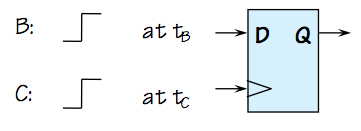Isn't this an Arbiter?
If we consider the application of our single-transition inputs to the D and clock of a flip flop as shown on the right to be an arbiter whose decision time is the flop's $t_{PD}$, we can argue convincingly that its Q output will conform to the arbiter specification when $t_B$ is sufficiently separated from $T_C$ that it falls outside of the setup/hold time window. Indeed, the specifications for the flip flop guarantee reliable operation so long as the dynamic discipline is met; thus choosing the $T_E$ of the arbiter spec to be sufficiently large as to cover the setup and hold times ensures that the device will output valid $0$ when the arbiter is required to output $0$, and valid $1$ when it is required to output $1$.

The only aspect of the arbiter spec that remains to be met is that, when $t_B$ and $T_C$ are sufficiently close (within $t_E$ of each other), the arbiter must produce either a valid $0$ or a valid $1$. The value is completely arbitrary, but it must be a valid logic level. Although the allure of the digital abstraction tempts us to assume that the flip flop output is valid outside of its bounded-time transitions, that assumption turns out to be a dangerous delusion. In fact, we forfeit the promise of output validity following a violation of the dynamic discipline by clocking an unsynchronized input.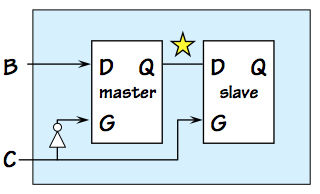Although the problem encountered by this arbiter implementation is fundamental to any arbiter implementation, we can gain some insight by diving in to see where our implementation fails. Recall that our D flip flop is constructed from two latches as shown to the left, and that on an active (positive) clock edge the slave "latches" a stored value that becomes the $Q$ output of the flop as described in Section 8.2.1.4.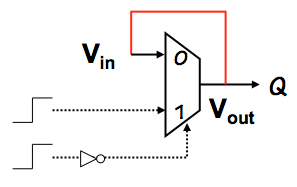The latched value actually circulates around in a self-sustaining cycle including a lenient multiplexor, as shown to the right as the feedback path in red. The other inputs to this multiplexor are such that the $V_{in}$ input is amplified to produce the more valid $V_{out}$ output voltage.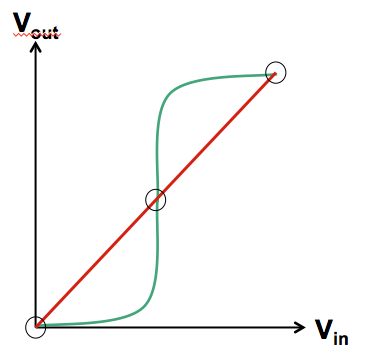The voltage domain representation of the multiplexor's role in this feedback loop is shown in green on the voltage transfer curve to the left, while the $V_{in} = V_{out}$ constraint imposed by the red feedback path is shown in red. These two curves represent two equations constraining the $V_{in}$/$V_{out}$ of the latch producing the output voltage of our flip flop; possible output voltages correspond to solutions to these simultaneous equations, represented graphically by intersections of the red and green curves.

We find three such intersections: two are the stable equilibria that represent lached valid $0$ and $1$ logic values. However, the geometry of our curves requires that there be a third intersection between these two, the unstable equilibrium often colloquially referred to as a meta-stable state. It represents a fixed point of the positive feedback path through the multiplexor -- an input voltage $V_M$ that causes that path to generate an output that is also $V_M$. Due to the continuity of the voltage transfer curve of the multiplexor, such a $V_M$ always exists. Typically $V_M$ is in the grey area between voltages which represent valid logic levels, representing neither a valid $0$ nor a valid $1$.

The bothersome metastable state is an inevitable consequence of bistable behavior, and is exhibited by familiar examples from our everyday lives. We recognise as an unlikely but real outcome of coin flips (landing on edge), horse races (dead heat), hockey games (overtime), and other real-world decision-making processes. The U.S. Presidential election of 2000 demonstrated that the fundamental difficulty of bounded-time decision making even extends to very large systems of arbitrary complexity.

The Metastable State

## 10.3. The Metastable State

We can observe several properties of the metastatble state:
1. If the dynamic discipline is obeyed (by observing flop setup/hold time constraints), the metastable state will be reliably avoided. It becomes a problem only when dealing with unsynchronized inputs.
2. The metastable voltage $V_M$ represents the switching threshold of our transistors, necessarily in the high-gain region of their operation. For this reason, it is normally not a valid logic level.
3. The metastable state represents an unstable equilibrium: a small perturbation in either direction will cause it to accellerate in that direction, quickly settling to a valid $0$ or $1$.
4. The rate at which the output voltage $V_{out}$ of a flop progresses toward a stable $0$ or $1$ value is proportional to the distance between $V_{out}$ and $V_M$.
5. Since $V_{out}$ is never exactly $V_M$, $V_{out}$ will always settle to a valid $0$ or $1$ eventually.
6. However, if $V_{out}$ may be arbitararily close to $V_M$, it may take arbitrarily long for $V_{out}$ to become valid.
7. The probabiliy of a flip flop remaining in a metastable state for $T$ seconds falls off exponentially with $T$; thus, simply waiting for a modest interval -- a clock cycle or two -- dramatically improves reliability.
Mechanical Analog

### 10.3.1. Mechanical AnalogMechanical Metastability
To gain some intuition about metastable behavior, let's explore a simple hypothetical bistable mechanical system. Consider a ball constrained to the confines of a deep well, whose floor has a modest hill that separates local low spots on the left and right sides of the well. Each of these low spots constitutes a stable equilibrium position for the ball, as shown to the right; however, there is an inevitable unstable equilibrium position at the top of the hill.

The device is bistable. We could in principle use the position of the ball -- left or right -- as a means to store a single bit, and arrange to change the value of the stored bit by kicking the ball to the left or right with enough force to reliably get it to the opposite side of the hill. Although we won't develop details of such a mechanical storage device here, it should be quite plausible to the reader that we could devise a way to make it work reliably. Of course, there would be some rules that must be followed for reliable operation; for example, one could not kick the ball to the left and to the right at about the same time. Such confusing instructions would compromise the momentum imparted to the ball, and might result in the ball landing near the unstable equilibrium point at the top of the hill -- the metastable state of our mechanical system.

Of course, given the vagueries of inaccuracies and noise, the ball is never precisely at the metastable point; it will always feel some slight force toward the left or the right. It will always roll down the hill to one of the stable equilibria eventually; but, depending on its distance from the metastable point, this trip may take an arbitrarily long time.

Observable Metastable Behavior

### 10.3.2. Observable Metastable Behavior

One reason for the reluctance of the digital community to accept the inevitability of arbitration failures is that they are inherently difficult to reproduce reliably in the laboratory. Even when violating the dynamic discipline by clocking unsynchronized data into a flip flop at high frequencies, observable metastable behavior is quite rare: it depends on pathological accidents of timing or other variables with precision beyond our ability to control them reliably.

However, it is reasonably straightforward to devise an experimental setup in which a flip flop is clocked repeatedly at high frequency, with a data input whose transitions are timed so as to maximize the probability of metastable failure.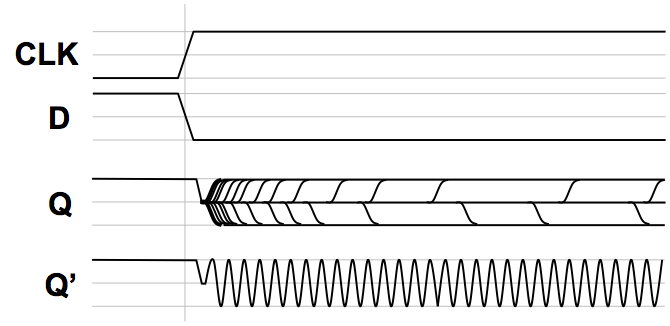Typical observed metastable behavior
We might use an oscilloscope to observe many such experiments, resulting in traces like those sketched to the right. The top traces of this image show the clock and D inputs to our test flip flop, making transitions at about the same time. The value labeled $Q$ shows the superposition of the output trace over many consecutive clock cycles. Note that in this family of traces, $Q$ assumes three dominant values: valid $0$, valid $1$, and the metastable voltage between the two. Following an active clock edge $Q$ moves to the metastable voltage, where it persists for a variable period before eventually snapping to a valid $0$ or $1$ value.

It should be noted that many other observable symptoms of arbitration failure are possible. Once we violate the dynamic discipline, there are no guarantees about the value circulating around our combinational feedback loop. The multiplexor input is invalid, so it need not produce a valid output. Rather than settling to the metastable voltage, for example, the value circulating might exhibit high-frequency oscillation such as that shown as $Q'$. Depending on parasitic reactive components in the feedback loop, such dynamic invalidity is sometimes observed.

Delay and Validity Probability

## 10.4. Delay and Validity Probability

We have already noted that problems with metastability can be avoided simply by assuring that the dynamic discipline is followed by our clocked circuits -- specifically, that input signals to each clocked component are valid and stable during that component's specified setup/hold time window. Although the problem is fundamental when an unsyncronized input must be passed to a clocked system, metastable behavior and its consequent system failures are still quite rare.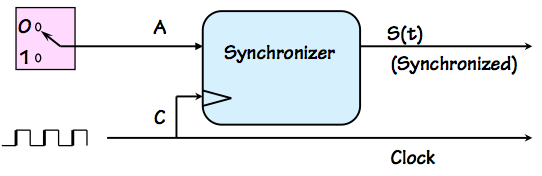Synchronizer test setup
Consider clocking a single unsynchronized transition into a flip flop whose setup and hold time are $t_S$ and $t_H$, respectively, and whose clock period is $t_{CLK}$. The probabability that an instantaneous transition will fall within the forbidden setup/hold time window, a prerequisite condition for arbitration failure, is thus $(t_S+t_H) / t_{CLK}$. This value represents a crudely pessimistic upper bound on the probability of a failure, and suggests that minimizing the $t_S+t_H$ window (by use of high-gain devices in our flip flop design) decreases probability of arbitration failures. It also implies that the problem is exacerbated by a high clock frequency.
Probability of nearness to metastable point

### 10.4.1. Probability of nearness to metastable point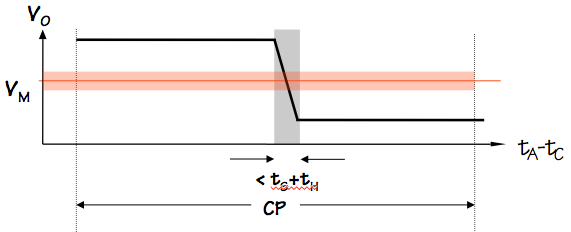In fact, metastable behavior is exhibited only when pathological input timing leads to a circulating voltage that is very close to the metastable value $V_M$. While a precise mathematical model for the circulating voltage $V_0$ immediately following an active clock edge is complex, we can again make the very conservative (pessimistic) assumption that it varies linearly between valid logic levels over the forbidden $t_S+t_H$ time window as represented by the black line in the graph above. In fact, problems occur only when this voltage falls near the metastable point, shown above as a narrow colored band centered on $V_M$.

Using this model, we can approximate the probability that $V_0$ -- the circulating voltage immediately following the clocking of an unsynchronized input -- falls within $\epsilon$ of the metastable voltage as \begin{equation} Pr[ | V_0 - V_M | \leq \epsilon ] \leq { ( t_S + t_H ) \over t_{CLK} } * { {2 \cdot \epsilon} \over ( V_H - V_L ) } \label{eq:prob_within_epsilon} \end{equation}

Delay cures metastability

### 10.4.2. Delay cures metastability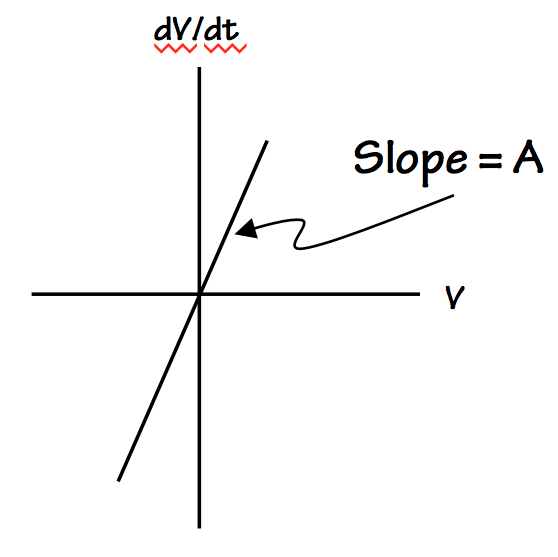Near the metastable point, our feedback loop amplifies the distance between the circulating voltage $V$ and the metastable point $V_M$, by moving $V$ away from $V_M$ at a rate proportional to $V - V_M$. The constant of proportionality $A$ is a function of circuit details like the amplification (gain), as well as the parasitic resistance and capacitance of the circuit. You may recognize this pattern -- rate X changes proportional to X -- as a formula for exponential growth, and indeed the trajectory of $V$ away from the metastable voltage is described by \begin{equation*} V - V_M \approxeq \epsilon \cdot e ^ { { T / \tau }} \end{equation*} in the vicinity of the metastable point, where $\epsilon$ is the starting distance $|V_0 - V_M|$ and $\tau$ is a time constant dependant on circuit parameters.

Given this model for the rate at which the circulating voltage $V$ progresses away from the troublesome metastable value, we can approximate the amount of time it will take for $V$ to progress from a value near $V_M$ to a valid $0$ or $1$. We do this by a formula that specifies, for a given time interval $T$, how close $V_0$ must be to $V_M$ to require $T$ seconds for $V$ to become valid: \begin{equation*} \epsilon ( T ) \approxeq (V_H - V_M) \cdot e ^ {-T / \tau} \end{equation*} Together with equation \eqref{eq:prob_within_epsilon}, we can approximate the probability that the output of our synchronizer flop remains invalid $T$ seconds after clocking an unsynchronized input as \begin{equation*} Pr_M(T) \approxeq Pr[ |V_0 - V_M| < \epsilon ( T ) ] \approxeq K \cdot e ^ {- T / \tau } \end{equation*} where $K$ and $\tau$ are constants derived from implementation parameters.

While we have glossed over a variety of issues in arriving at this formula, it underscores an important observation about the metastable behavior that causes synchronization failures: the probability that a flipflop output will remain invalid decreases exponentially with elapsed time. This key fact allows us an engineering workaround, which reduces the unsolvability of the arbitration problem from a showstopper to an annoyance: we can, in fact, clock unsynchronized inputs to an arbitrarily high level of reliability by simply introducing modest delay for any metastable behavior to settle.

Average time
T$Pr_M(T)$between failures
$31$ ns$3 \cdot 10^{-16}$$1 year 33.2 ns3 \cdot 10^{-17}$$10$ years
$100$ ns$3 \cdot 10^{-45}$$10 ^ {30}$ years
To appreciate the scale of the delays involved, the table to the right shows some failure probabilities computed using conservative values from decades-old process parameters. While the actual numbers are of little relevence, it is noteworthy that the exponential relationship between delay and settling time yields astronomically high reliability rates from modest delays. You may recall that the age of the earth is estimated to be on the order of $5 \cdot 10^9$ years, making the failure rates attainable at the cost of tens of nanoseconds delay seem quite acceptable.
Bounded-time Discrete Mapping

## 10.5. Bounded-time Discrete Mapping

The problem of making "which came first?" decisions in bounded time confronted by the arbiter is, in fact, an instance of a more general difficulty: the problem of mapping a discrete variable into a continuous one. If the mapping is nontrivial (in the sense that its range includes multiple discrete values), there will be input values $i_1$ and $i_2$ that map to distinct discrete outputs $V_1$ and $V_2$, respectively. If the mapping is to be performed by a mechanism that computes $V = f(i)$ for some continuous function $f$, the continuity of $f$ assures that there will be input values between $i_1$ and $i_2$ that produce output values between $V_1$ and $V_2$. Indeed, even if $f$ has a discontinuity for some input $i_1 < i_M < i_2$, the mapping of $i_M$ is ambiguous and hence problemmatic.

We have seen this problem in the mapping of continuously-variable voltages to discrete $1$s and $0$s in Chapter 5, where we conspired to avoid the difficult decision problems by an engineering discipline that excludes a range of voltages from our mapping and avoids requiring our circuits to interpret these values as valid logic levels. When we moved to discrete time in Chapter 8, we introduced an analogous discipline to duck hard problems deciding the ordering between active clock edges and input data transitions. In this case, our excluded "forbidden zone" is the range of continuously variable timing relationships ruled out by the setup/hold time window of the dynamic discipline.
What we cannot do reliably

### 10.5.1. What we cannot do reliably

The difficult problems identified in this chapter share the attributes that they involve a nontrivial continuous-to-discrete mapping that must be made in bounded time. If we relax either of these constraints, solutions become plausible. The astute reader might recognise that this is is essentially the same problem, translated to the time domain, that was addressed in the voltage domain in Chapter 5 by the forbidden zone and noise margins. The static discipline avoids unbounded-time decisions about whether a continuously variable voltage represents a $1$ or a $0$; the dynamic discipline avoids unbounded-time decisions about whether a clock transition at $t_C$ comes before a data transition at $t_D$. Each of the avoided problems involves a mapping of a continuous variable into two discrete cases: in the former case the variable is a voltage, in the latter a time interval $t_C - t_D$.We have already seen that the asynchronous arbiter, which decides the ordering of two transitions within a finite propagation delay following either (the first, the last, or a specific input) cannot be reliable if its implementation follows the continuous mathematics of classical physics; this fact remains true even if we allow it to report an arbitrary result in close cases. The synchronizer of Section 10.1 is a minor variant of the arbiter, and suffers similar unreliability issues.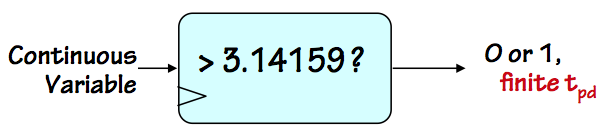Other continuously variable inputs also resist knife-edge decisions in bounded time. Consider a module that compares an input voltage to a fixed threshold, reporting if the input is above or below the threshold value. Even if we allow it to be wrong in close cases, this analog comparator cannot make a decision in bounded time; again, this confronts the fundamental difficulty of a bounded-time continuous-to-discrete mapping.
What we CAN do reliably

### 10.5.2. What we CAN do reliably

Although nontrivial bounded-time mapping of continuous to discrete variables confronts fundamental problems, slight relaxation of critical problem constraints makes seemingly close relatives tractible.

Perhaps the most obvious solvable variant is our clear ability to make a reliable device that performs a trivial mapping of continuous to real values in bounded time. A device implemented by a connection to ground, for example, could reliably report that $T_B < T_C$ for any relative timing of two transitions, or that $V < 3.14 volts$ for any input voltage $V$.A more interesting example is an unbounded-time arbitor such as the one shown to the left. Rather than requiring that this device produce a valid $S$ output within a prescribed $t_{PD}$, it is allowed to take any (finite) interval to make its decision, and signal (via a $Done$ output) when its decision is made. Given reasonable specifications (include a range of input timings for which either answer is acceptable), this device can operate reliably.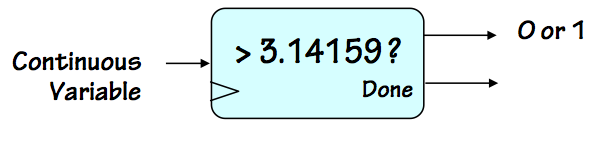Similarly, an unbounded time analog comparator can in principle be made to work reliably. Again, a finite range of allowable errors must be provided for in its specification.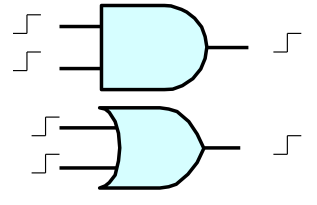Although bounded-time which-came-first decisions are hard, certain functions of signals with asynchronous transitions are straightforward. Consider a 2-input device which signals an output a finite period after the second of two input transitions has occurred; can such a device be made reliable? How about signaling shortly after the first of the two transitions? These specifications are nicely met by combinational AND and OR gates.
Folk Cures

### 10.5.3. Folk Cures

The fundamentals of synchronization and arbitration failures have taken decades to be accepted by the engineering community. This history is laced with interesting proposed "solutions" to the problem of building a reliable synchronizer, most of which can be viewed with the same skepticism we might apply to a new proposal for a perpetual motion machine.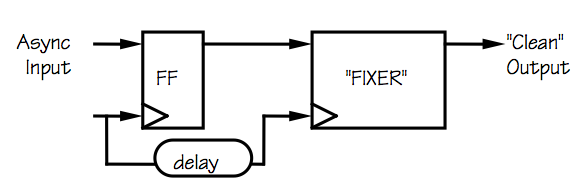One class of naive proposals involves a fixer module that can be spliced into specific circuit locations that are experiencing synchronization failures, such as that shown to the right. The input to the fixer module is a potentially invalid logic level, such as the output from a flip flop clocking an asynchronous input. The fixer is supposed to detect whether its input is a valid logic level and correct it (to a valid level) if it is invalid, all within a bounded time interval.

While there are many things wrong with such proposals, one that is obvious in the restrospect of decades of recent history is that the problem of deciding whether a given voltage is valid cannot itself be solved in bounded time. Once again, this decision represents a nontrivial mapping of a continuous variable (input voltage) to a discrete one (validity); there will always be an input value that will produce an output halfway between true and false.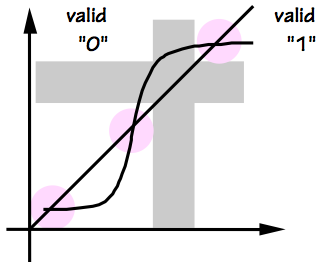One particularly creative class of proposals involves eliminating the problem by an auspicious manipulation of our definitions of logical validity. While accepting the need for noise margins and forbidden zones in our mapping of voltages to valid logic levels discussed in Chapter 5, it is not actually required that the metastable fixed-point voltage $V_M$ of a flip flop fall in the forbidden zone. We might devise a logic family, for example, where the metastable voltage is deemed a valid $0$. In this family, a flip flop that clocks an unsynchronized input may still hang at the metastable voltage for an arbitarily long interval; but now its output will be a valid $0$ during that interval.

The downside of this approach? After clocking an unsyncronized input, a flip flop whose output is metastable (producing what is now interpreted as a valid $0$) will eventually progress to a valid logic level. About half the time, it will choose to emerge from the metastable state to become a valid $1$. In our new logic family, this will be seen by surrounding logic as a spontaneous transition of the flop output from $0$ to $1$ -- a behavior not likely to be appreciated by the circuit designer.

Dealing with Asynchronous Inputs

## 10.6. Dealing with Asynchronous Inputs

The fundamental difficulty associated with clocking asynchronous signals is an accepted fact among the modern engineering community. Failure to obey the dynamic discipline creates a finite probability of an invalid logic level in our digital system. One invalid signal may stimulate others: the static discipline guarantees valid outputs from valid inputs, but guarantees nothing for invalid inputs. In principle, a requirement to accept asynchronous inputs threatens the integrity of our digital system engineering discipline.

Once understood, however, the practical impact of the synchronization problem is modest. Keys to our coping with asynchrony include the following:

1. Synchronize clocks: Avoid the problem where possible, by using a single clock discipline or synchronizing the clocks of separate subsystems that must communicate.
2. Delay: Where asynchronous inputs cannot be avoided, allow sufficient delay for the output of the synchronizing flip flop to settle that the probability of failure is acceptably low. The exponential decay of failure probability with delay interval reduces the problem of failure to a fairly modest cost in latency.
3. Hide delay: Under certain circumstances, enginnering tricks can be used to eliminate some or all of the delay by predicting arbitration problems and solving them in advance of the actual input event.
The first of these guidelines simply reflects the fact that there is a cost associated with synchronization of input data, and that synchronization problems shouldn't be casually introduced -- for example, by needless use of multiple unsynchronized clocks in different parts of a system. While there are circumstances where a multiclock system makes sense, such a system is carefully partitioned into several clock domains and special care given to communication between the domains.

The second guideline reflects our recognition that we can reduce the probability of failure to an arbitrarily low level by adding modest delay.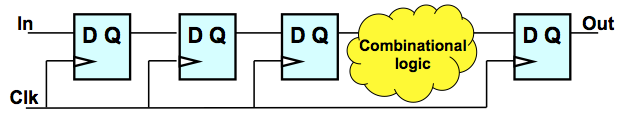Often one sees a configuration such as that shown to the right, forcing an input signal to pass through multiple, apparently redundant, flip flops. This pattern represents an effective way to convert an asynchronous input to a derived synchronous signal whose probability of invalidity $t_{PD}$ after an active clock edge is acceptably small.

A common informal analysis of this circuit reasons that if the probability that the output of the leftmost flop is invalid is some small number $p$, the probability of invalidity at the output of the flop to its right is $p ^ 2$, $p^3$ at the third flop, etc. While there may be some merit to this reasoning, the more general principle is the exponential falloff of failure probability with delay outlined in Section 10.4.2. Each flop the signal passes through delays its entering subsequent circuitry by a clock cycle, and consequently enhances its reliability by a large factor. Under most circumstances, the delay of an asynchronous input by a few clock cycles reduces failure probability to a ridiculously low level with negligible impact on the function or performance of the system.

In latency-sensitive applications, however, the delay required for reliable communication may compromise performance. In these cases, it may be worth exploring some special-case optimizations suggested by the third of our guidelines. For example, communication between digital subsystems that require different frequency clocks might be made reliable if one clock period is a multiple of the other that maintain a fixed synchronous relationship.This idea may be generalized to deal effectively with clocks whose periods have the ratio $m/n$ for various integral $m$ and $n$. 1 The predictablity of the timing of active clock edges in such cases allows the generation of a "scrubbed" version of the local clock for use in clocking in asynchronous data. The scrubbed clock omits active clock edges that come at dangerous times. While this decision -- whether a given clock edge is dangerous -- is itself an abitration problem, it can be performed beforehand in real time or even precalculated and wired into the design.

In general, any known constraints on the timing of the incoming asynchronous input can be exploited -- at some engineering cost -- to reduce or eliminate the delay needed to reliably communicate.

Chapter Summary

## 10.8. Chapter Summary

Synchronization failures have played an unexpectedly significant role in the history of digital engineering, likely due in large part to the conceptual appeal of the digital abstraction. Digital engineers, seduced by the comfortable world of $1$s and $0$s, have demonstrated a remarkable resistance to the notion that the cost of violating the dynamic discipline is abdicating the guarantee of validity. And once any invalid signal creeps into our system, it can propagate elsewhere: the "valid in $\rightarrow$ valid out" construction rule no longer prevents logically invalid levels.

Important facts to understand and remember:

• Synchronization failure is a problem only when the dynamic discipline is violated, e.g. by an input that changes asynchronously.
• Every bistable storage device has, in addition to the two stable equilbria used to represent its two possible values, a third unstable equilibrium called the metastable state. Without constraints on the timing of input changes (the dynamic discipline), the bistable device may enter this state, resulting in invalid output values;
• Metastable behavior eventually resolves to a valid $1$ or $0$, but the time required is unbounded.
• The probability of metastable behavior persisting for $t$ seconds falls off exponentially with $t$, so a modest delay after an unsynchronized input can reduce the probability of invalid outputs to be negligible.
• Synchronization failures are best avoided by
1. Avoiding asynchronous inputs where possible; and
2. Allowing sufficient delay after clocking unavoidably asynchronous inputs.
The primary practical impact of these issues is the acceptance by the digital engineering community of modest delay as a fundamental cost associated with asynchronous inputs.